# Measuring LTE Uplink EVM and In-Band Emissions

EVM is computed based on the received signal constellation error as the difference between the ideal received waveform and the measured waveform for allocated resource blocks. The in-band emission is defined as the ratio of the user equipment (UE) output power in a non-allocated resource block (RB) to the UE output power in an allocated RB. LTE System Toolbox™ can measure EVM and in-band emissions.

With these measurements, you can assess the quality of the received signal and the transmission channel. These measurements are key to assessing the quality of an LTE transmitter, including any impairment caused by RF and analog components.

This example shows the five steps for using LTE System Toolbox to perform EVM and in-band emission on an uplink signal per TS 36.101 Annex F .

A UE transmitted waveform is created using a reference measurement channel (RMC) and random physical uplink shared channel (PUSCH) data. To generate the waveform, we use the `lteRMCULTool` app of LTE System Toolbox as shown in Figure 1. We set the appropriate parameters such as the reference channel identifier and redundancy version (RV) sequence. Then we provide variable names for the transmitted output waveform, the resource grid, and the RMC configuration. And, finally, we click Generate waveform.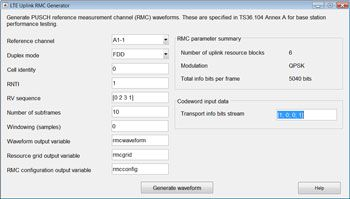Figure 1. Waveform generation using lteRMCULTool app.

Alternatively, we use the MATLAB® function `lteRMCUL` to first create a configuration structure for given UE settings specific to a given fixed reference channel (FRC). This structure is used in conjunction with the `lteRMCULTool` function and random PUSCH data to generate the waveform and resource grid. The following four lines of MATLAB code perform these operations and allow direct access to customize a reference waveform:

```frc = lteRMCUL('A3-1'); % UE Configuration, a TS36.101 FRC
frc.PUSCH.RVSeq = 0;    % Redundancy version
data = randi([0 1], frc.PUSCH.TrBlkSizes(1), 1);  % Create random PUSCH data
[txWaveform, txGrid] = lteRMCULTool(frc, data);  % UE transmitted waveform generation
```

## Impairment and Noise Modeling

In an actual situation, this signal is typically fed to the RF circuit for amplification, up-conversion, and transmission over the air. This step typically introduces impairments in signal quality due to imperfect amplifiers, modulators, and so forth, which is why it is crucial to measure the signal EVM after this step.

At this point, we add impairments to the transmitted waveform to simulate a device under test. For example, we introduce impairments to model:

• 1.2% transmit EVM modeled with additive noise
• 33 Hz frequency offset
• A constant 0.01 – 0.005j I/Q imbalance offset

The MATLAB script below shows how we can easily add these types of impairments with LTE System Toolbox functions.

```% Model transmit EVM with additive noise
info = lteSCFDMAInfo(frc);  % Obtain FFT length from FRC structure
txEVMpc = 1.2;        	  % Set desired transmit EVM in percent
gain = txEVMpc/(100*sqrt(double(info.Nfft))); % Compute gain of additive noise
evmModel = gain * complex(randn(size(txWaveform)), randn(size(txWaveform)))/2;
rxWaveform = txWaveform+evmModel; 	     % Add noise to the transmitted waveform
foffset = 33.0;  	    	  % Frequency offset in Hertz
rxWaveform = lteFrequencyCorrect(frc, rxWaveform, -foffset);
iqoffset = complex(0.01, -0.005);
rxWaveform = rxWaveform+iqoffset;
```

The received waveform must undergo timing synchronization and I/Q offset correction before we can compute EVM and in-band emissions. On a subframe-by-subframe basis, we must also estimate frequency offsets and accordingly correct the received waveforms. The MATLAB script below shows how to perform timing synchronization and I/Q offset correction using functions of LTE System Toolbox.

Note: Frequency offset correction is not shown in the MATLAB code below and is executed within the `hPUSCHEVM` helper function featured next in Measuring EVM.

```% Synchronize received waveform timing_offset =
lteULFrameOffset(frc, frc.PUSCH, rxWaveform); rxWaveform =
rxWaveform(1+timing_offset:end, :);
% Preform IQ offset correction
iqoffset = mean(rxWaveform);
rxWaveform = rxWaveform - iqoffset;
```

## Measuring EVM

The received waveform is subject to EVM measurement subframe-by-subframe. To compute EVM, we need to reconstruct ideal symbols in addition to measured received symbols of the allocated resource blocks. Ideal symbols are obtained by a combination of the following operations: single-carrier frequency division multiple access (SC-FDMA) demodulation to obtain the received resource grid, channel estimation, PUSCH equalization, symbol demodulation, and decoding followed by recoding the received bits, rescrambling, and remodulation. Figure 2 shows the process of EVM measurement.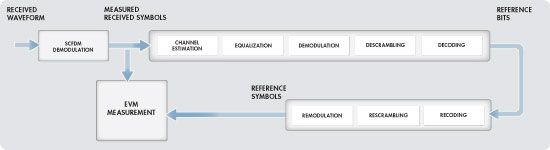Figure 2. Process of EVM measurement.

The average EVM is measured at two locations in time (low and high), where the low and high locations correspond to alignment of the Fast Fourier transform (FFT) window within the start and end of the cyclic prefix (CP). Figure 3 shows the locations of low and high measurement points within one single-carrier frequency division multiplexing (SC-FDM) data symbol. In FFT samples, the difference between the low and high locations is known as EVM window length.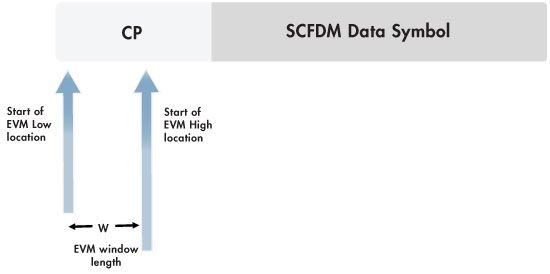Figure 3. Locations of low and high EVM measurement points.

EVM window length depends on channel bandwidth or whether or not a normal CP is used. Table 1 illustrates dependency of EVM window length on bandwidth for normal CP. LTE System Toolbox requires low and high locations to be specified as a fraction of the CP length.

 EVM Parameters Bandwidth (MHz) 1.4 3 5 10 15 20 FFT size 128 256 512 1024 1536 2048 Number of resource blocks 6 15 25 50 75 100 EVM window length (W) (in FFT samples) 5 12 32 66 102 136

Table 1. An excerpt of 3GPP TS 36.101 V8.8.0, Annex F, Table F.5.3.-1  for normal cyclic prefix.

All of these computations are performed within the single helper function `hPUSCHEVM` of LTE System Toolbox. The MATLAB code below shows how to call the function by providing as inputs the FRC and the received waveform `rxWaveform`, and obtain as outputs the average overall PUSCH EVM and the average overall demodulation reference signal (DRS) EVM for allocated resource blocks.

```% Compute EVM and in-band emissions
[evmpusch, evmdrs] = hPUSCHEVM(frc, rxWaveform);
% Display EVM results
fprintf('Averaged overall PUSCH EVM: %0.3f%%\n', evmpusch.RMS*100);
fprintf('Averaged overall DRS EVM: %0.3f%%', evmdrs.RMS*100);
```
```Averaged overall PUSCH EVM: 1.627%
Averaged overall DRS EVM: 0.925%
```

Note that the EVM of each E-UTRA carrier for QPSK/BPSK and 16QAM modulation should not exceed the EVM level of 17.5% and 12.5% respectively, per 3 GPP TS36.101, Table 6.5.2.1.1-1 . The results obtained in our measurement above is well within this range.

## Measuring In-Band Emissions

To measure in-band emissions, we need to compute UE output power in a non-allocated RB. The number of non-allocated RBs depends on the FRC we select. By running the following MATLAB code, we can visualize the allocated and non-allocated RBs within the transmission bandwidth.

```surf(1:size(txGrid, 2), 1:size(txGrid, 1), 20*log10(abs(txGrid(:, :, 1))));
title('Visualizing the allocated and non-allocated RBs for inband-emissions');
xlabel('Slot index');
ylabel('Subcarrier index');
zlabel('Symbol power');
```

Figure 4 shows a single allocated RB (RB=0) to the right, which extends 12 subcarriers and 5 non-allocated RBs to the left of the allocated RB. The first adjacent RB outside the allocated RB is denoted by RB=1, and other non-allocated resource blocks are shown in sequence to a maximum of RB=5.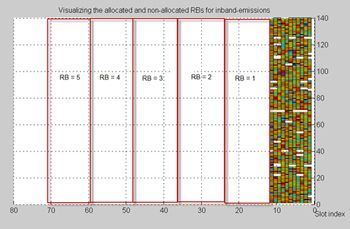Figure 4. Allocated RBs and non-allocated RBs within the 1.4 MHz BW for our example FRC.

All computations related to separating allocated and non-allocated RBs and computing in-band emission are performed within the same helper function hPUSCHEVM of LTE System Toolbox. The MATLAB scripts below show how to call the function with the same input arguments as described in the EVM section. We then obtain, as its third output argument, the absolute and relative in-band emissions measurements per slot.

```% Compute EVM and in-band emissions
[~, ~, emissions] = hPUSCHEVM(frc, rxWaveform);
% Plot the absolute in-band emissions
figure;
h = plot(emissions.Absolute.', '*-');
title('Absolute in-band emissions for each unallocated RB', ...
'FontSize', 10, 'FontWeight', 'normal');
xlabel('Slot', 'FontSize', 10, 'FontWeight', 'normal');
ylabel('Absolute in-band emissions', 'FontSize', 10, 'FontWeight', 'normal');
nRB = length(emissions.DeltaRB);
s = cell(1, nRB);
for k=1:nRB
s{k} = sprintf('\\Delta _ R _ B=%d', emissions.DeltaRB(k));
end
legend(h, s, 'Location', 'Best');
```

The above script also plots Figure 5 below, which visualizes the absolute in-band emissions for each allocated RB, ranging from the RB adjacent to the allocated RB (RB=1) and the furthest non-allocated RB from the allocated RB (RB=5).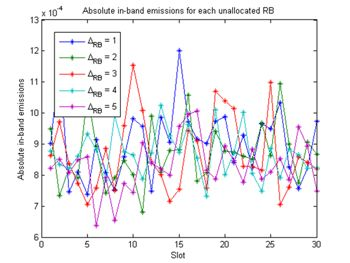Figure 5. Absolute in-band emissions as a function of slot index.

## Summary

LTE System Toolbox enables you to perform EVM and in-band emissions measurement in accordance with standard documents TS  36.101 Annex F  and TS 36.104 Annex E .

This capability is a key enabler if you design RF components for an actual implementation of an LTE system because you can quickly  assess performance of your system according to 3GPP specifications.

The measurement workflow presented takes minimal time to define and execute. Also, access to MATLAB code opens unlimited design modifications and access to algorithms used. LTE System Toolbox includes downlink  and uplink  EVM measurements.

## References

 3GPP TS 36.101 – User Equipment Radio Transmission and Reception
 3GPP TS 36.104 – Base Station Radio Transmission and Reception
 PDSCH Error Vector Magnitude (EVM) Measurement
 LTE Uplink EVM and In-Band Emissions Measurements

Published 2015 - 80712v00